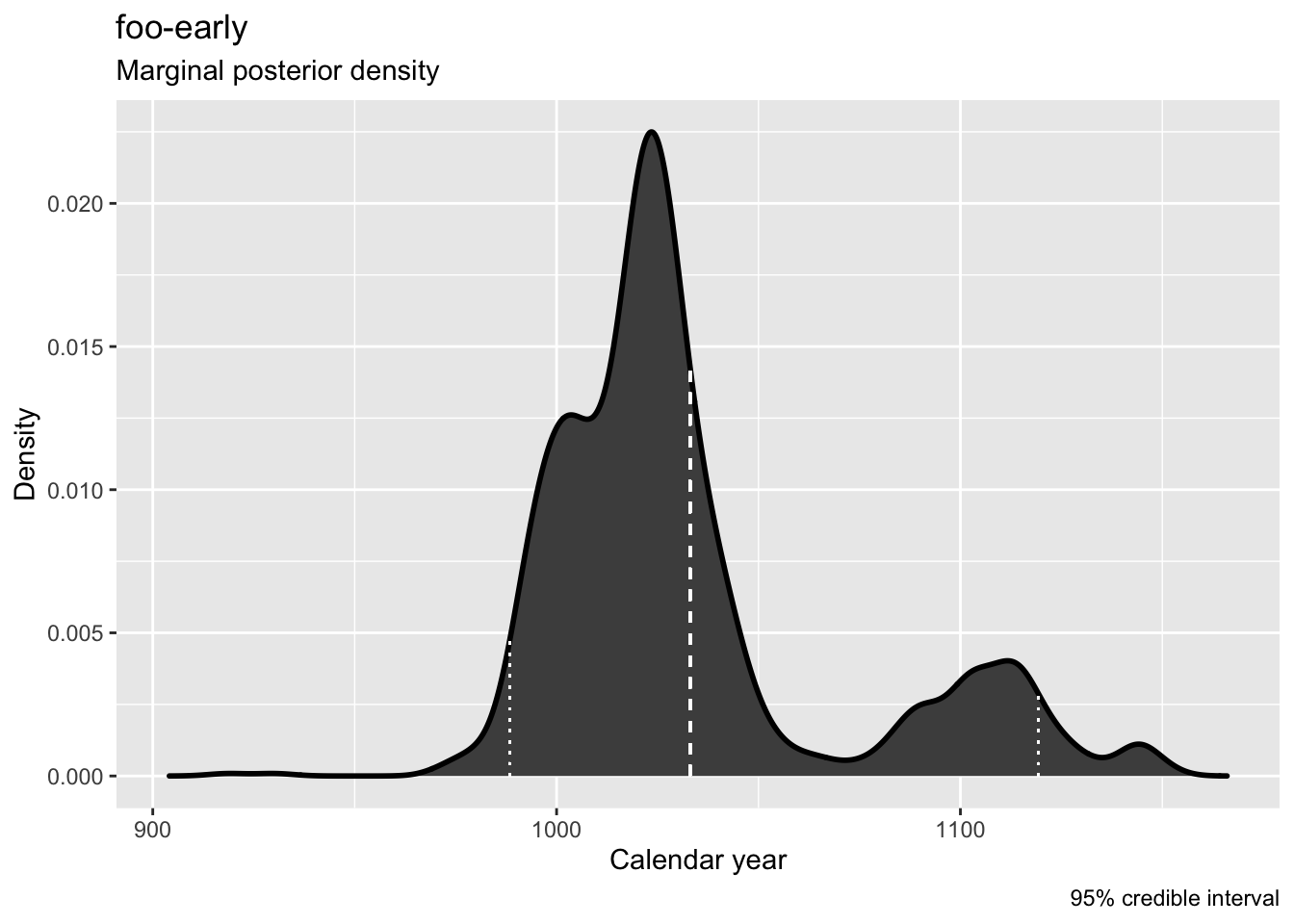# 4. ArchaeoPhases : Reproducibility

## Introduction

Version `ArchaeoPhases 1.5` adds new read, plot, and statistical functions designed to encourage reproducibility. This vignette describes some of these functions and illustrates how to use them to reproduce an analysis.

New read functions `read_bcal()`, `read_oxcal()`, and `read_chronomodel()` are intended to replace the general purpose function, `ImportCSV()`. The new functions are built on the function `read_csv()`, which is fast and able to read remote files, as well as local files. The new functions return S3 objects that can identify the file that produced them. This facility might be useful in situations where an analysis is based on a remote file that isn’t under the analyst’s control, or when files are shared electronically and potentially subject to corruption.

## Test for Original Data File

The following code block illustrates this capability. After the `ArchaeoPhases` package has been loaded, the `read_oxcal` function is used to read a remote `OxCal` file and assign an S3 object to the variable `oxc`. The `original_file` method of the `oxc` object checks if the original file used to create it has changed since the object was created. If the original file still exists and is unchanged, then the function returns `TRUE`. If the original file cannot be found, or has changed, then the function returns `FALSE`.

``````## load ArchaeoPhases
library(ArchaeoPhases)
data(oxc)
## returns TRUE, if ox.csv has not changed on the server
original_file(oxc)``````
`` TRUE``

## New Plot Functions

The new plot functions, `multi_dates_plot()`, `tempo_activity_plot()`, `tempo_plot()`, `marginal_plot()`, `multi_marginal_plot()`, and `occurrence_plot()` are functional replacements for the originals with camelCase names, e.g., `TempoPlot()` -> `tempo_plot()`. They return S3 objects with `plot()` and `reproduce()` methods that inherit from data frame and can be passed to statistical functions.

The following code block illustrates the `plot()` and `reproduce()` methods. The call to the `marginal_plot` function draws a plot of the first marginal posterior in the `oxc` object and returns an S3 object, which is assigned to the variable `oxc.mar`. The call to the `plot` method of the `oxc.mar` object draws the same plot and also returns an S3 object. Note that the S3 objects returned by `marginal_plot()` and `plot()` differ because the calls that created them differ. Nevertheless, the data returned by the two calls are identical, as expected. The call, `reproduce(oxc.mar)` checks that the original file is accessible and has not changed, then recreates the plot. If successful, the object it returns is identical with the object it reproduces.

``````## create mariginal plot object
oxc.mar <- marginal_plot(oxc)````````````## use plot method to reproduce marginal plot
oxc.mar.plot <- plot(oxc.mar)``````